Analogy

Analogy means 'similarity' or ‘correspondence’. i.e., having similar features.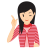Few Important things to Remember

In question based on analogy, a particular relationship between words/letters/numbers is given and another similar relationship has to be identified from the alternatives provided.

Types of Analogy

Analogy may be broadly divided into the following types:

1. Word Analogy
2. Number Analogy
3. Alphabet Analogy
4. Mixed Analogy

1. Word Analogy

This type of analogy covers every type of relationship that one can think. There are many ways of establishing a relationship like quantity and unit, worker and tools, cause and effect, word-synonym, word-antonym, country and capital, state and capital, country and currency, animal and its young one (kid), male and female, animal and its resting place, game and place of playing, occupation, the working place and the work, study and topic etc.

In word analogy, the candidates are required to discover the relationship between question pair and then to find the required pair of words that is most similar to that relationship.

Direction (Example Nos. 1)In this question, there is a certain relationship between two given terms on one side of (::) and one term is given on another side (::) while another term is to be found from the given alternatives, having the same relation with this term as the terms of the given pair bear.

Example 1: Choose the correct alternative.

Newspaper : Press :: Cloth : ?

(a) Tailor                    (b) Fibre

(c) Mill                        (d) Textile

Solution:  (c) As, 'Newspaper' is prepared in 'Press', in the same way, 'Cloth' is manufactured in the 'Mill'.

2. Number Analogy

In this type of analogy, numbers are given in a pair of group on one side. The candidate has to find the relationship between them and then find the number or group of numbers which will replace the question mark in the other pair on other side.

The relationship in number analogy can be of following types

• Even and odd numbers

• Multiplication and division of numbers

• Addition and subtraction of numbers

• Square and cube roots of numbers, etc.

Direction (Example Nos. 1-3)In the following questions, select the related number pair from the given alternatives.

Example 1: Choose the best alternative.

23 : 13 : : 54 : ?

(a) 40    (b) 41    (c) 44    (d) 39

Solution:  (c) Firstly, we will check the relation between first pair i.e. 23 and 13

We can see, 23 - 10 = 13
Similarly, the number in the place of ? will be
54 - 10 = 44

Example 2: Choose the best alternative.

14 : 225 : : 17 : ?

(a) 250    (b) 340    (c) 300    (d) 324

Solution:  (d) Firstly, we will check the relation between first pair i.e. 14 and 225

We can see, (14 + 1 )2 = (15)2 = 225
Similarly, the number in the place of ? is obtained as
(17 + 1)2 = (18)2 = 324

Example 3: Choose the best alternative.

20 : 11 : : 102 : ?

(a) 49    (b) 52    (c) 65    (d) 85

Solution:  (b) Firstly, we will check the relation between first pair i.e. 20 and 11

We can see, (20/2 + 1 ) = 10 + 1 = 11
Similarly, the number in the place of ? is obtained as
(102/2 + 1) = 51 + 1 = 52

3. Alphabet Analogy

In this type of analogy, there is a relationship between the given alphabets or group of letters. The candidate has to remember the position of letters in English alphabetical order and find out the relationship between the given letters or group of letters in the first pair and then find a set of letters to fit in place of question mark in second pair. The relationships between the letters can be of following types

• Vowels or consonants

• Increasing/decreasing position of letters.

• Reverse order of position of letters.

• Skipped letter relation

Here, following table is showing letter's position in English alphabetical series.

Forward Alphabet Backward
1 A 26
2 B 25
3 C 24
4 D 23
5 E 22
6 F 21
7 G 20
8 H 19
9 I 18
10 J 17
11 K 16
12 L 15
13 M 14
Forward Alphabet Backward
14 N 13
15 O 12
16 P 11
17 Q 10
18 R 9
19 S 8
20 T 7
21 U 6
22 V 5
23 W 4
24 X 3
25 Y 2
26 Z 1

Direction (Example Nos. 1-3)Choose the word / letter(s) which will replace the question mark

Example 1:  AFKP : BGLQ : : CHMR : ?

(a) DINS    (b) DNIS    (c) DFKP    (d) DJOT

Solution:  (a) Firstly, we will check the relation between first pair i.e. AFKP and BGLQ ,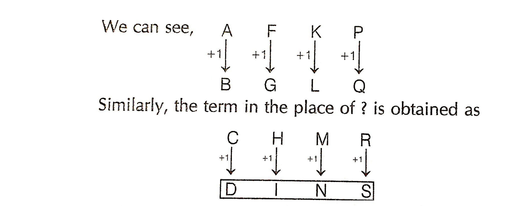Example 2:  SNOP : ONSP : : CLAY : ?

(a) ALCY                                    (b) LCYA

(c) LYCA                                    (d) ACLY

Solution:  (a) Firstly, we will check the relation between first pair i.e. SNOP and ONSP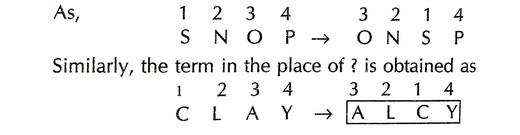Example 3:  ASTN : ZTSO : : MSUB : ?

(a) LTTC                                  (b) LRRC

(c) LTTA                                  (d) LRRO

Solution:  (a) Firstly, we will check the relation between first pair i.e. ASTN and ZTSO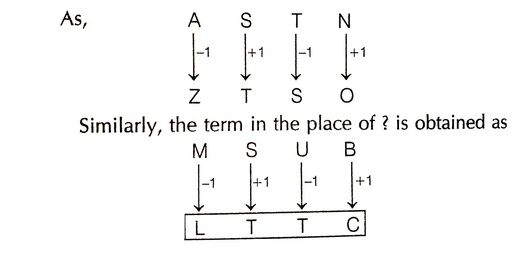4. Mixed Analogy

In this type of analogy, there is a certain relationship between the given group of letters and numbers on one side. The candidates are asked to find out the correct alternative based on the given relationship. The relationship in mixed analogy can be of following types

• The position of the alphabets or letters

• Sum or product of the position of alphabets or letters etc.

Direction (Example Nos. 1-2)Choose the number which will replace the question mark.

Example 1:  NEWS : 14, 5, 23, 19 : : PAPER : ?

(a) 16, 5, 16, 1, 18              (b) 18, 5, 16, 1, 16

(c) 16, 1, 16, 5, 18              (d) 32, 2, 32, 10, 36

Solution:  (c)Each letter assigned its position from left in English alphabet.

So, PAPER ----> 16, 1, 16, 5, 18

Example 2:  FILM : 10 : : HOTEL : ?

(a) 12                                  (b) 15

(c) 18                                  (d) 30

Solution:  (a)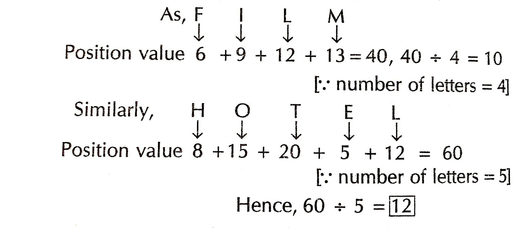Types of questions

Different types of question based on analogy that are asked in various competitive exams, have been given below

Type #1: Direct/Simple Analogy

In this type of analogy, two elements are given which are related to each other in a particular manner and other element is given followed by four alternatives. Finally, the candidate is required to identify the relationship between the first two elements. Then, the candidate is required to pick that element from the alternatives which bear exactly the same relationship to the third element, as the first two bear.

Example 1: 'College' is related to 'Student' in the same way as 'Hospital' is related to

(a) Doctor                                      (b) Nurse

(c) Medicine                                  (d) Patient

Solution:  (d) In the 'College', education is given to 'Students' in the same way, treatment is given to the 'Patient' in 'Hospital'.

Example 2: 'Major' is related to 'Lieutenant' in the same way as 'Squadron Leader' is related to

(a) Group Captain                      (b) Flying Attendant

(c) Flying Officer                         (d) Pilot Officer

Solution:  (c) 'Major' and 'Squadron Leader' are equivalent ranks in the Army and the Air Force, respectively. Same as 'Lieutenant' and 'Flying officer' are equivalent ranks in the Army and Air force, respectively.

Type #2: Completing/Choosing the Analogous Pair

In this type of questions, two components are given, which are related to each other in a particular way. The candidate is required to find out the relationship between the first two components and choose the component/pair from the given alternatives, which have the same relationship as there in between the first two.

Direction (Example Nos. 1-2)In each of the following questions, select the related word / letters group from the given alternatives.

Example 1:  Boat : Oar : : Bicycle : ?

(a) 20 : 105                     (b) 22 : 100

(c) 18 : 190                     (d) 15 : 225

Solution:  (a) Second denotes that part of the first, on which the effort is applied to move it.

Example 2:  FLOWER : REWOLF : : FRUITS : ?

(a) STUIRF                                  (b) STUIFR

(c) STIURF                                  (d) STRUIF

Solution:  (c) In first pair, second term is the reverse order of first term.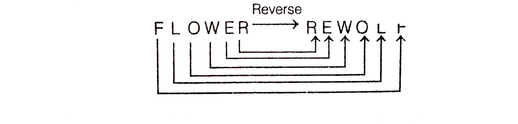Similarly, in second pair,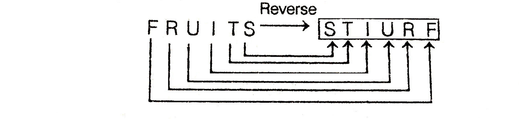Direction (Example Nos. 1-2)The following questions, consist of two words / numbers having a certain relationship to each other, following by same pair of words / numbers select the related pair that has the same relationship as the original pair of words.

Example 1:  Pigeon : Peace : : ?

(a) Crown : Head           (b) White Flag : Surrender

(c) Laurels : Victory       (d) War : Liberty

Solution:  (b) As, 'Pigeon' is a symbol of 'Peace'.

Similarly, 'White Flag' is symbol of 'Surrender'.

Example 2:  9 : 50 : : ?

(a) 20 : 105                  (b) 22 : 100

(c) 18 : 190                  (d) 15 : 225

Solution:  (a) In first pair, we have relation between 9 and 50.

as (9 x 5) + 5 = 45 + 5 = 50

Similarly, (20 x 5) + 5 = 100 + 5 = 105

Type #3: Choosing a Similar Word/Number

In this type of questions, a group of three words/numbers is given, followed by four alternatives. The candidate is required to choose the alternative, which is similar to the given group of word/numbers.

Direction (Example Nos. 1-2)In each of the following questions, choose that set of number / word which has the same relationship among the given the three numbers / words.

Example 1:  144, 256, 324

(a) 625                    (b) 175

(c) 188                    (d) 189

Solution:  (a) The given numbers are perfect squares.

As, 144 = (12)2; 256 = (16)2; 324 = (18)2

Similarly, 625 = (25)2

Example 2:  Bhilai, Durgapur, Bokaro

(a) Baroda                         (b) Chennai

(c) Chandigarh                 (d) Rourkela

Solution:  (d) All cities are famous for steel plants.

Type #4: Multiple Word/Number Analogy

In this analogy, a group of three inter-related words/numbers is given. The candidate is required to trace out the relationship among these words/numbers and choose another group with similar relationship from the given alternatives.

Direction (Example Nos. 1-2)In each of the following questions, choose that set of numbers/words from the four alternatives sets that is similar to the given set.

Example 1:  Pen : Pencil : Ink

(a) Orange : Banana : Juice

(b) Table : Chair : Wood

(c) Cow : Milk : Curd

(d) Fish : Shark : Water

Solution:  (a) 'Pen', 'Pencil' belong to the same category i.e. stationary and pen contains 'Ink'.

Similarly, 'Orange' and 'Banana' belong to the same category i.e. fruits and orange contains 'Juice'.

Example 2:  Given set (6, 10, 16)

(a) 9, 12, 18

(b) 5, 10, 15

(c) 6,11,12

(d) 7,11,17

Solution:  (d)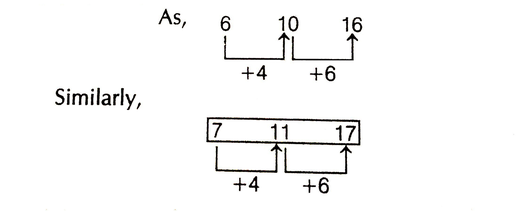LearnFrenzy provides you lots of fully solved "Analogy" Questions and Answers with explanation.